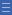## Featured resource

Home > Topdrawer > Fractions > Good teaching > Equivalence > Grids and arrays

# Grids and arrays

Grids and arrays

Using grids and arrays to represent fractions begins to focus attention on the multiplicative relationships between pairs of equivalent fractions.

The most valuable aspect of using grids and arrays is that they focus thinking on factors and multiples, which is the essential foundation for developing general strategies for creating equivalent fractions.

Representations such as circles and fraction walls are more likely to promote additive thinking.

For example, this fraction wall clearly shows that $$\frac{3}{6}$$ is equivalent to $$\frac{1}{2}$$ but tends to suggest the additive relationship of $$\frac{1}{6}$$ + $$\frac{1}{6}$$ + $$\frac{1}{6}$$ = $$\frac{1}{2}$$.

On the other hand, having to create the one grid that will show both halves and sixths demands thinking about the multiplicative relationship between the denominators 2 and 6 (i.e. 2 $$\times$$ 3 = 6).

The aim of activities with grids and arrays is to develop a conceptual understanding of the processes for recognising and creating equivalent fractions.

Students need time to explore these processes with models rather than simply learning an abstract procedure for forming equivalent fractions.

Yes

Yes

Name Class SectionOverlay grids Folder 17Dynamic fractions Folder 17Year 4: Investigate equivalent fractions used in contexts Infobox 3Year 6: Compare fractions with related denominators and locate and represent them on a number line Infobox 3Source Infobox 3